# number 5 LULILULUI. E. 5. (10 points) A uniform board of a length 6 m supported...

number 5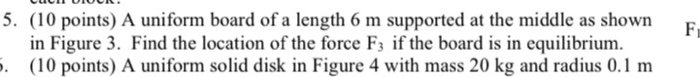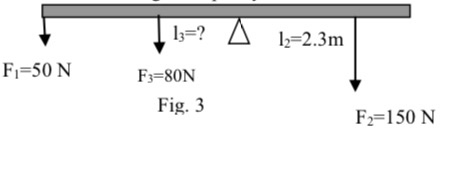LULILULUI. E. 5. (10 points) A uniform board of a length 6 m supported at the middle as shown in Figure 3. Find the location of the force Fz if the board is in equilibrium. 6. (10 points) A uniform solid disk in Figure 4 with mass 20 kg and radius 0.1 m
12=2.3m Fi=50 N | 1;=? A F3=80N Fig. 3 F2=150 N

Bar is supported at the middle so

l1 = distance of force 1 = 3 m

now given that bar is in equilibrium so moment balance about support

l1 * F1 + l3 * F3 = l2 * F2

3 * 50 + l3 * 80 = 2.3 * 150

l3 = 2.4375 m

feel free to drop comment if you have any doubt.

#### Earn Coin

Coins can be redeemed for fabulous gifts.

Similar Homework Help Questions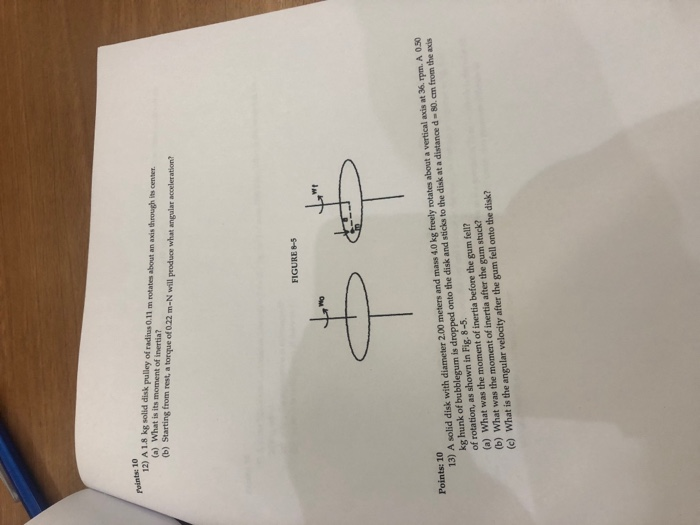Please help Points: 10 12) A 18 kg solid disk pulley of radius 0.11 m rotates about an axis through its center (a) What is its moment of inertia? (b) Starting from rest, a torque of 0.22 m-N will produce what angular acceleration? FIGURE 8-5 13) A solid disk with diameter 2.00 meters and mass 4.0 kg freely rotates about a vertical axis at 36.rpm. A 0.50 kg hunk of bubblegum is dropped onto the disk and sticks to the...

• ### Problem 5: A 5 kg uniform serving table and 1 kg bowl is supported by a...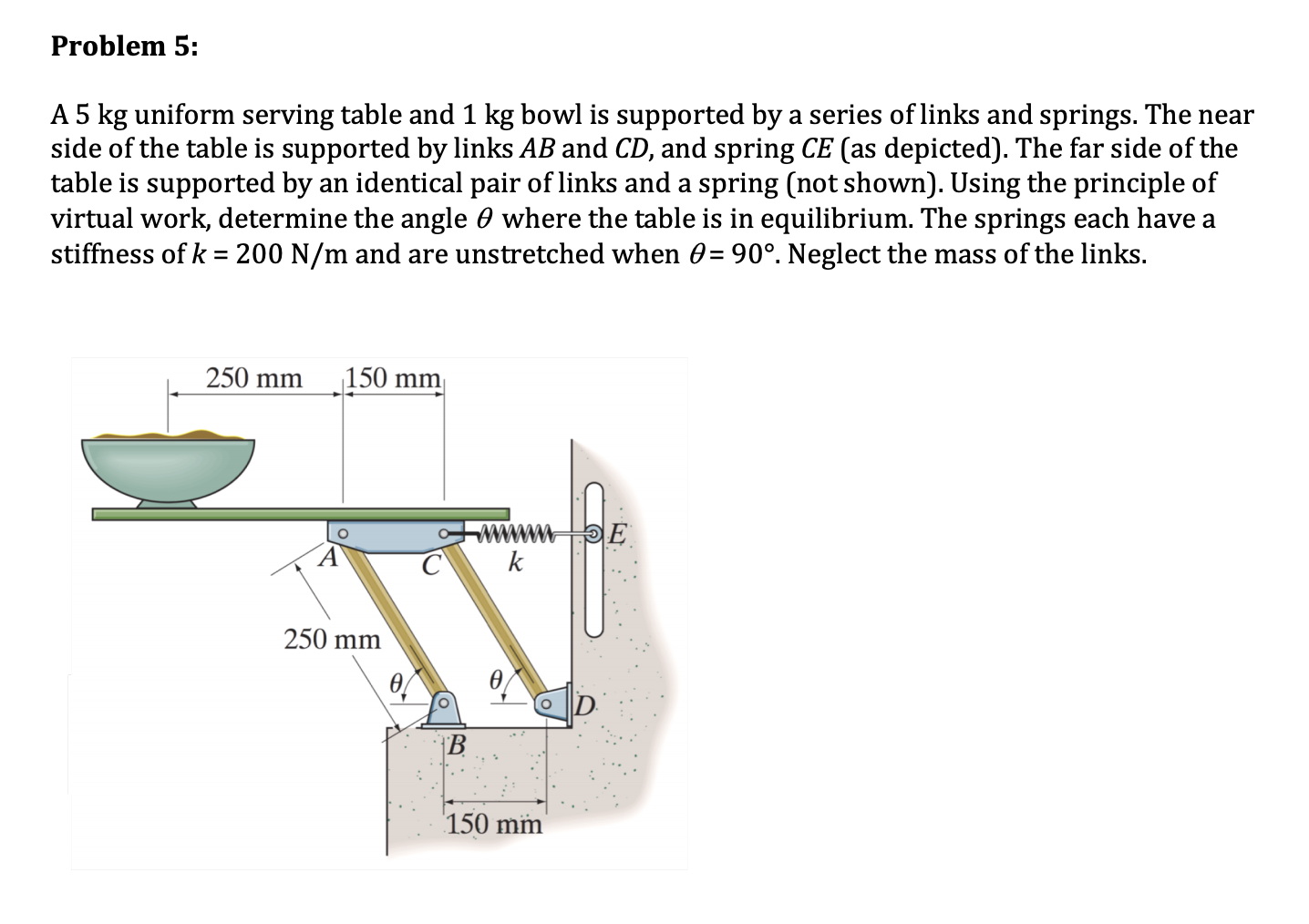Problem 5: A 5 kg uniform serving table and 1 kg bowl is supported by a series of links and springs. The near side of the table is supported by links AB and CD, and spring CE (as depicted). The far side of the table is supported by an identical pair of links and a spring (not shown). Using the principle of virtual work, determine the angle o where the table is in equilibrium. The springs each have a stiffness...

• ### 6. A uniform rod of length 5.0 m and weight 60.0 N is supported at its...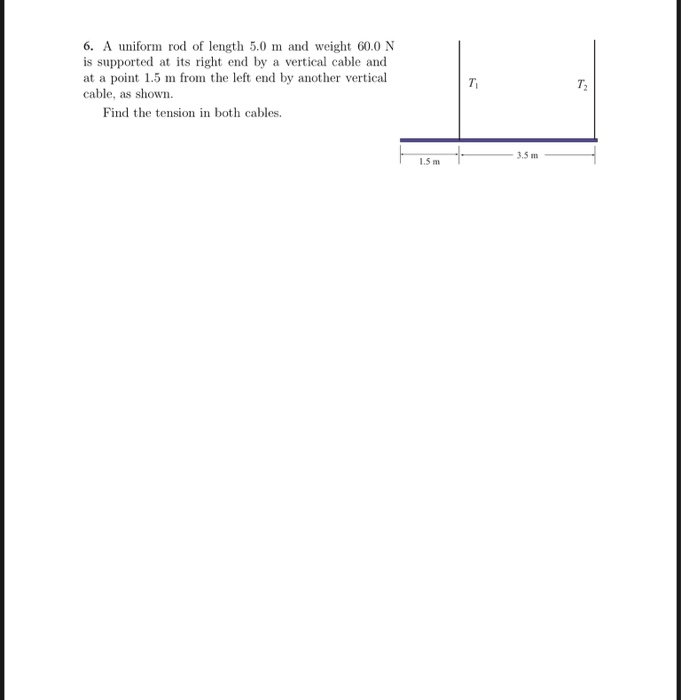6. A uniform rod of length 5.0 m and weight 60.0 N is supported at its right end by a vertical cable and at a point 1.5 m from the left end by another vertical cable, as shown. Find the tension in both cables. Sm 3.5 m

• ### Please answer all parts of question (a-c). 4:45 PM 33% AT&T Notes May 1, 2019 at 4:45 PM Problem 1 a) A uniform beam (5.00 m long, 40.0 kg) is attached to the wall and supported by a cab...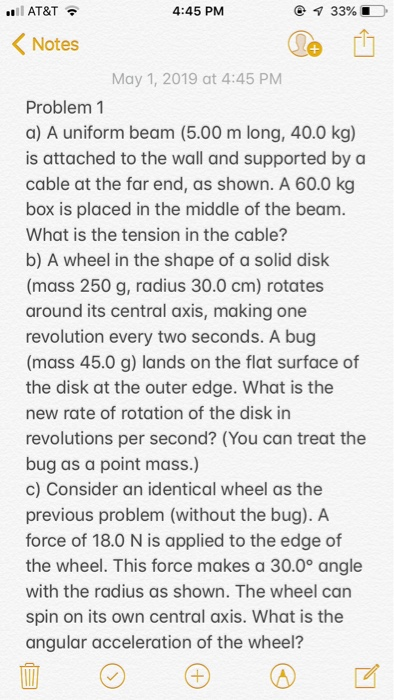Please answer all parts of question (a-c). 4:45 PM 33% AT&T Notes May 1, 2019 at 4:45 PM Problem 1 a) A uniform beam (5.00 m long, 40.0 kg) is attached to the wall and supported by a cable at the far end, as shown. A 60.0 kg box is placed in the middle of the beanm What is the tension in the cable? b) A wheel in the shape of a solid disk (mass 250 g, radius 30.0 cm)...

• ### Figure Q5(b) shows a frame ABCDE that is supported by a hinge at A and E...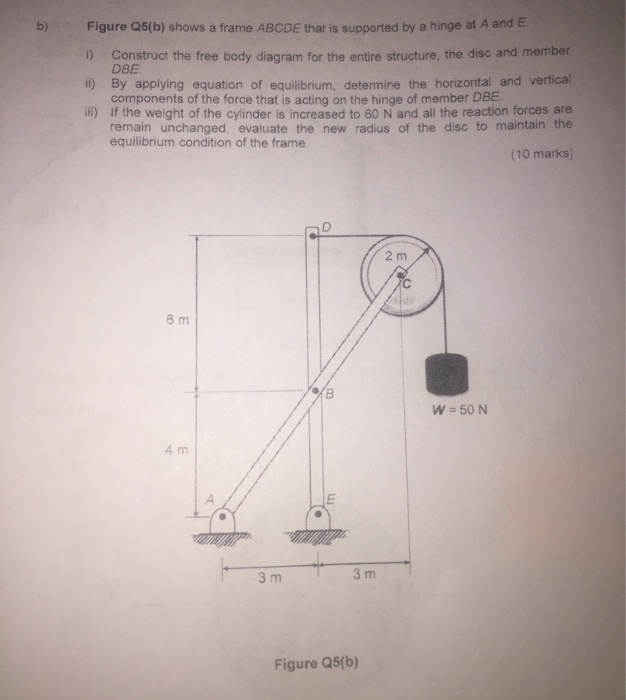Figure Q5(b) shows a frame ABCDE that is supported by a hinge at A and E b) ) Construct the free body diagram for the entire structure, the disc and member DBE t) By applying equation of equilibrium, determine the horizontal and vertical components of the force that is acting on the hinge of member DBE il) if the weight of the cylinder is increased to 60 N and all the reaction forces are remain unchanged evaluate the new radius...

• ### PlOBlem 4 (10 points) 2 m 3 m The frame is made up of members ACE, BCD and DE. The frame is pin- 6 m supported at A...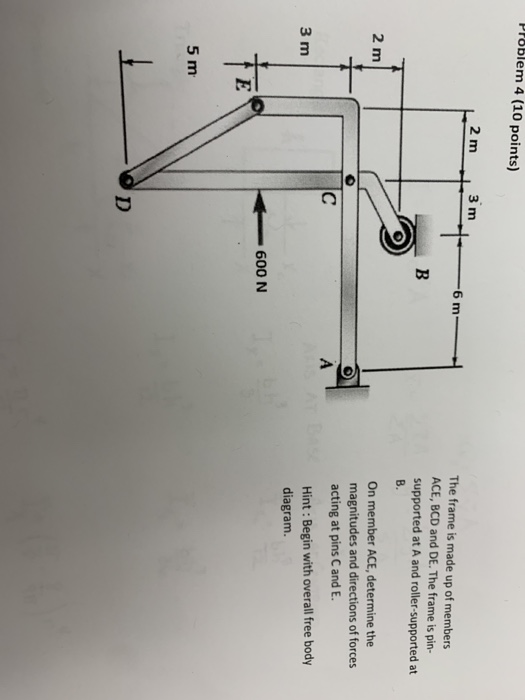PlOBlem 4 (10 points) 2 m 3 m The frame is made up of members ACE, BCD and DE. The frame is pin- 6 m supported at A and roller-supported at B. On member ACE, determine the 2 m magnitudes and directions of forces acting at pins C and E Hint: Begin with overall free body 3 m diagram 600 N 5 m PlOBlem 4 (10 points) 2 m 3 m The frame is made up of members ACE, BCD...

• ### Question 6 (2 points) Consider a diving board of length 7.0 m. The right end of...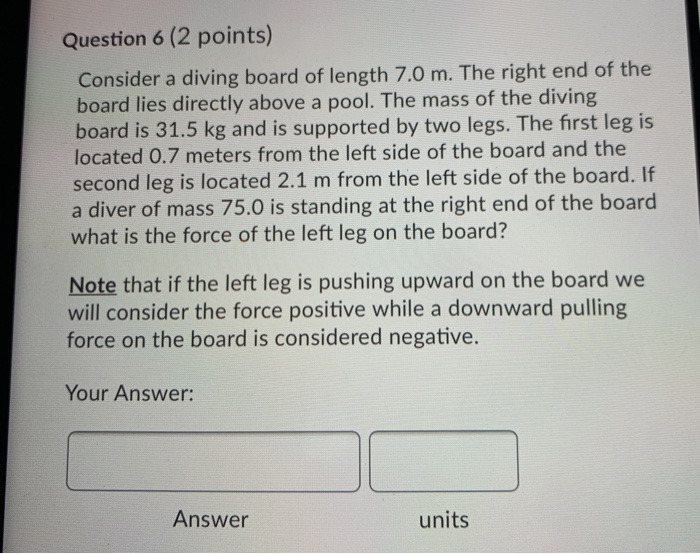Question 6 (2 points) Consider a diving board of length 7.0 m. The right end of the board lies directly above a pool. The mass of the diving board is 31.5 kg and is supported by two legs. The first leg is located 0.7 meters from the left side of the board and the second leg is located 2.1 m from the left side of the board. If a diver of mass 75.0 is standing at the right end of...

• ### Please show all work with algebra. disk 5. A uniform disk of mass M and radius...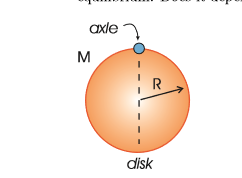Please show all work with algebra. disk 5. A uniform disk of mass M and radius R is suspended from an axle at its rim, as (a) (9 points) Draw a free body diagram for the disk when it is pulled out by a (b) (9 points) Write out the torque equation for small oscillations of the disk about shown in the figure below. It is then pulled out by a small angle and released small angle. its equilibriumi position...

• ### 3) Two people carry a heavy electric motor by placing it on a uniform board, m...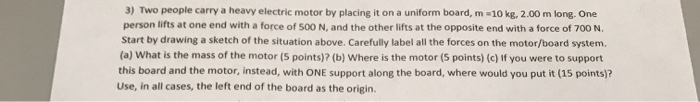3) Two people carry a heavy electric motor by placing it on a uniform board, m 10 kg, 2.00 m long. One person lifts at one end with a force of 500 N, and the other lifts at the opposite end with a force of 700 N Start by drawing a sketch of the situation above. Carefully label all the forces on the motor/board system (a) What is the mass of the motor (5 points)? (b) Where is the motor...

• ### 6. -2 points PSES 12.P046. My Notes A 1150 N uniform boom is supported by a...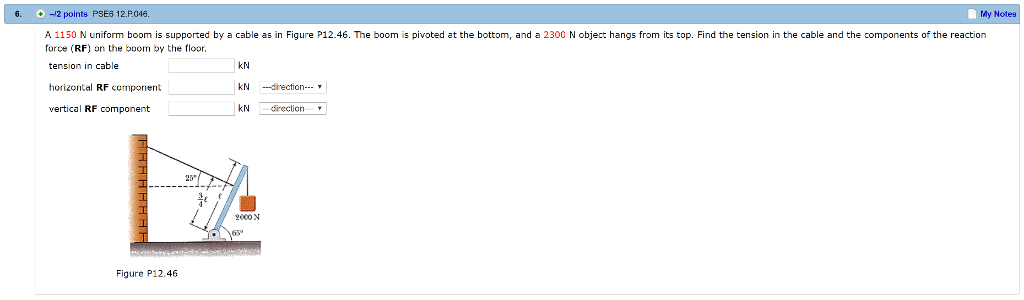6. -2 points PSES 12.P046. My Notes A 1150 N uniform boom is supported by a cable as in Figure P12.46. The boom is pivoted at the bottom, and a 2300 N object hangs from its top. Find the tension in the cable and the components of the reaction force (RF) on the boom by the floor kN tension in cable horizontal RF component vertical RF component KN kNirection 20 2000N 65 Figure P12.46

Free Homework App